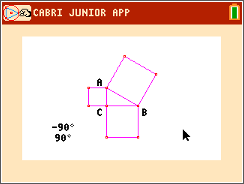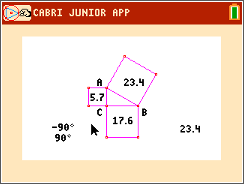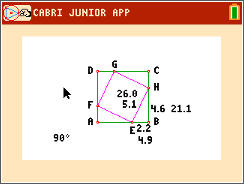••• ##### Device
• TI-84 Plus
• TI-84 Plus Silver Edition
•TI-84 Plus C Silver Edition
•TI-84 Plus CE
• ##### Report an Issue

Geometry: The Pythagorean Theorem
by Texas Instruments#### Overview

Students will construct figures that prove the Pythagorean Theorem in two different ways.

#### Key Steps

•In Problem 1, students use Cabri™ Jr. to prove the Pythagorean Theorem by constructing a triangle bounded by three squares. Students find the areas of each square. They also find the sum of the two smaller squares. Students will notice that the sum is equal to the area of the largest square hence proving the theorem.

•In Problem 2, students construct a square and then they construct a quadrilateral inside the square. They will prove that the inscribed quadrilateral is a square algebraically then confirm their proof by applying Pythagorean Theorem to one of the triangles.